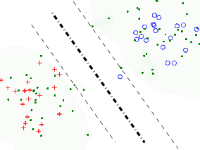Machine learning is a field of artificial intelligence, which use empirical data to extract characteristic of interest of their unknown underlying probability distribution. Knowledge about a given problem is learnt from examples (training data). This knowledge is implicit, unlike explicit knowledge that can be given by formal definitions or rules. A machine learning algorithm is evaluated on its generalization capability, which is its ability to apply successfully the learnt knowledge to unseen data. Different kinds of machine learning algorithms exist, depending on the goal. Supervised algorithms such as support vector machine (SVM) are used for classification tasks while unsupervised algorithms such as manifold learning algorithms are used to extract the relation between the examples.

# Manifold learning (dimensionality reduction)

In machine learning, the data are usually represented in a high dimensional feature space. Nevertheless in practice, the data are restricted to a limited area of the feature space. This leads to the well known problem of the curse of dimensionality. The manifold learning techniques, also known as dimensionality reduction aim to find a mapping of the data from the high dimensional feature space to a new space of lower dimensions. The manifold learning methods estimates the geometry of the dataset locally, around each data point.

# Training LS-SVM Classifier in semi-supervised mode

The learning process typically assumes some form of a priori knowledge of the contextual problem at hand in the form of examplar data associated with labels. These data, called the training set, are used to design a classifier, the performance of which is measured on a separate dataset, called the testing set. This is supervised learning in which the performance of the classifier on the test set is viewed as an estimate of the true performance of the system (i.e. the performance on the whole space).